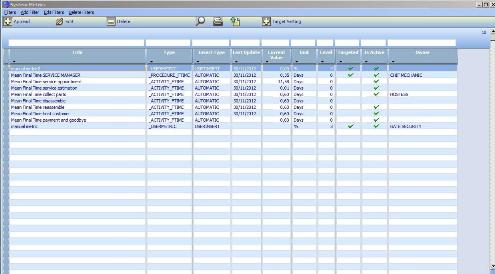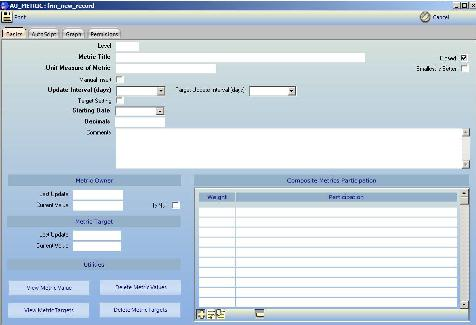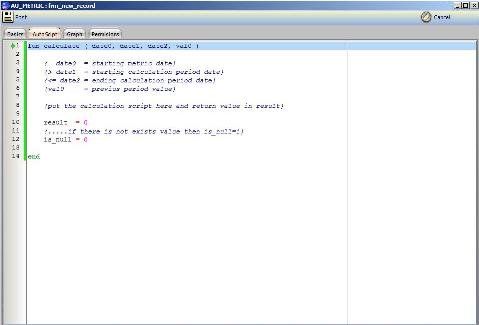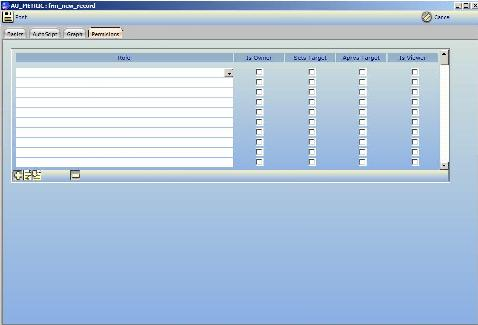# How to Insert a Metric

This article explains how to insert a Metric into a Mykosmos/BOS server.
See the steps below.

1. Open the application.
2. Go to menu "System/Metric Definitions" and activateThe form that shows all the Metric definitions

3. Click on button "Append" and the form for the new Metric shows. This is a multipage one.In this page, we put the script for the periodic Metric calculationIn this page, we set the owner role of the Metric and the viewers (as roles) for the Metric

In the first page, we put "Title", "Unit Measure", "Update Period", "Decimal Points", "Starting Date" of the metric. There is a possibility that the Metric is to be provided manually by users. In this case, we check the "Manual Insert".

In this first page, there is the possibility to define a composite Metric. A composite Metric is one that consists of the weighted participation of other Metrics of the lower level. This way is possible to construct Balanced Scorecards of various depth. The Metrics that can participate in a composite Metric are always targeted ones.

In the second page, we provide the script that calculates the periodic value of Metric. An example script is the following:

fun calculate ( date0, date1, date2, val0 )

{  date0  = starting metric date}
{> date1  = starting calculation period date}
{<= date2 = ending calculation period date}
{val0     = previus period value}

start_sql "*" "KOSMOS"
SELECT SUM(VALUE) AS SVALUE FROM SALES
WHERE (DATE > :D1) AND (DATE <= :D2)
end_sql
q = QueryByName()
call TSetParam(q,"D1",date1)
call TSetParam(q,"D2",date2)
call TExecute(q)
val = TGetFld(q,"SVALUE")
count   = TRecordCount(q)
if (count = 0) then
is_null = 1
else
result = val
is_null = 0
endif
call FreeEmbSQL(q)

end

The required variables in the script are "result" and "is_null".

In the third page, we provide the role that is "owner" for the Metric. So if the period of Metric is "1 Month" then every month an A
ctivity will be issued to the user's terminal for the inspection of the Metric value. Viewers of the Metric also can be defined in this page. Every viewer of the Metric can see it in the "KPIs" section of his/her User Interface.